## Orthogonal Matrix

A matrix is orthogonal if the transpose is equal to its inverse , that is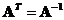.

Since computing matrix inverse is rather difficult while computing matrix transpose is straightforward, orthogonal matrix make difficult operation easier. Orthogonal matrix is important in many applications because of its properties.

Example:

Is matrix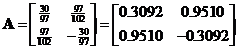an orthogonal matrix?

To test whether a matrix is an orthogonal matrix, we multiply the matrix to its transpose. If the result is an identity matrix, then the input matrix is an orthogonal matrix.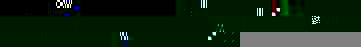Thus, matrix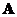is an orthogonal matrix.

To create random orthogonal matrix as in the interactive program below, I created random symmetric matrix and compute the modal matrix from concatenation of the Eigen vectors .

The interactive program below is designed to answers the question whether the given input matrix is an orthogonal matrix. When you click Random Example button, it will create random input matrix to provide you with many examples of both orthogonal and non-orthogonal matrices. You can also try to input your own matrix to test whether it is an orthogonal matrix or not.

## Properties

Some important properties of orthogonal matrix are

• Orthogonal matrix is always a square matrix
• Ifis an orthogonal matrix order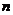with real entries then
• The inner product of two row vectors or two column vectors of matrixis zero
• The row vector and the column vector of matrixis orthonormal (the Euclidean vector norm is one)
• The transpose matrix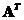is also an orthogonal matrix
• The inverse matrix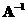is also an orthogonal matrix
• The product to its transpose is identity matrix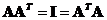• The absolute magnitude the determinant is one, that is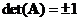• The eigenvectors ofare real and orthogonal
• The eigenvalues ofare equal to +1 or -1 (note the theoretical of eigenvalue and eigenvectors of orthogonal matrix is sometimes difficult to obtain numerically due to round off error).
• If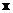and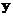are vector ofdimensions, then
• Inner product with orthogonal matrix is preserved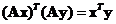• Euclidean norm is preserved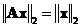• For any square matrix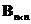, there exist a unitary matrix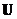such that matrix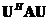is upper triangular (Schurs theorem).
• Unitary matrix is generalization of orthogonal matrix with entries of complex numbers
• Both Hermitian and Unitary matrix (including symmetric and orthogonal matrix) are called normal matrix because the eigen vectors form orthonormal set.